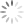夜凉如水

# 基于Python的机器学习框架scikit-learn学习笔记01

2017-11-14 周二 晴 北京 清华大学 开始
2017-11-19 周日 阴 北京 清华大学 完成

## 1 scikit-learn是什么?

scikit-learn官网：http://scikit-learn.org/

scikit-learn（简称sklearn）是一个基于Python语言的机器学习框架，这个东西好不好我也不太清楚。我也是看到助教和课上有同学说可以用这个来做作业，才决定了解下它的。

## 2 安装scikit-learn

``````pip install –U xxx.whl
``````

``````xxx_amd64.whl is not a supported wheel on this platform.
``````## 3 scikit-learn的使用

### 3.1 BaggingClassifier

BaggingClassifier定义：

``````class sklearn.ensemble.BaggingClassifier(base_estimator=None, n_estimators=10, max_samples=1.0, max_features=1.0, bootstrap=True, bootstrap_features=False, oob_score=False, warm_start=False, n_jobs=1, random_state=None, verbose=0)
``````

base_estimator 基学习器 None，对应决策树
n_estimators 基学习器个数 10 int型
max_samples 样本抽取个数或比例 1.0 整数对应个数，小数则对应比例
max_features 特征抽取个数或比例 1.0 整数对应个数，小数则对应比例
bootstrap 样本抽取是否放回 True bool型
bootstrap_features 特征抽取是否放回 false bool型
oob_score 是否用bag外样本估计误差 bool型

``````import numpy as np
from sklearn.ensemble import BaggingClassifier

# 加载样本数据
X = dataset[:,0:6]
y = dataset[:,6]

# 训练分类器
bagging = BaggingClassifier()
bagging.fit(X, y)

# 测试：用训练好的分类器分类
xt = [[ 3., 2., 5.,7., 8., 6.],
[ 3., 2., 5.,7., 8., 7.]]
yt = bagging.predict(xt)
print(yt)
``````

### 3.2 RandomForestClassifier

RandomForestClassifier定义：

``````class sklearn.ensemble.RandomForestClassifier(n_estimators=10, criterion=’gini’, max_depth=None, min_samples_split=2, min_samples_leaf=1, min_weight_fraction_leaf=0.0, max_features=’auto’, max_leaf_nodes=None, min_impurity_decrease=0.0, min_impurity_split=None, bootstrap=True, oob_score=False, n_jobs=1, random_state=None, verbose=0, warm_start=False, class_weight=None)
``````

n_estimators 基学习器个数 10 int型
max_features 特征抽取个数或比例 “auto” 整数对应个数，小数则对应比例; “auto”:sqrt(n_features); “sqrt”:sqrt(n_features)； “log2”：log2(n_features)； None：n_features.
criterion 生成决策树时使用的标准 “gini” “gini”或”entropy”
bootstrap 样本抽取是否放回 True bool型
oob_score 是否用bag外样本估计误差 bool型

``````import numpy as np
from sklearn.ensemble import BaggingClassifier
from sklearn.ensemble import RandomForestClassifier

# 加载样本数据
X = dataset[:,0:6]
y = dataset[:,6]

# 训练RF分类器
rf = RandomForestClassifier()
rf.fit(X, y)

# 测试：用训练好的分类器分类
xt = X[0:2,:]
yt_rf = rf.predict(xt)
print(yt_rf)
``````

``````class sklearn.ensemble.AdaBoostClassifier(base_estimator=None, n_estimators=50, learning_rate=1.0, algorithm=’SAMME.R’, random_state=None)
``````

base_estimator 基学习器 决策树分类器
n_estimators 基学习器个数 50 int型
learning_rate 学习率？不懂 1 float
algorithm 算法 ‘SAMME.R’ {‘SAMME’, ‘SAMME.R’}
random_state 与随机数生成有关 none int型

11.18日解决问题：自己胡乱调，胡乱试了两天，发现需要设置AdaBoostClassifier的 基分类器参数`DecisionTreeClassifier`，虽然默认就是`DecisionTreeClassifier`，但是如果不手动写上，分类的错误率非常低。调整`DecisionTreeClassifier`的深度`max_depth`有效果。

``````import numpy as np
from sklearn.tree import DecisionTreeClassifier
import time

# 加载样本数据
nClasses = 17
nFeatures = 6
X = dataset[:,0:6]
y = dataset[:,6]
nSamples = len(y)

maxDepth = 12
begin = time.clock()
end = time.clock()
print('\nElapsed time: %.8f seconds\n' % (end-begin) )

# 测试：用训练好的分类器分类
xt = X[0:2,:]
print(xt)
print(yt)
``````

### 3.4 利用sklearn生成训练样本数据

`make_classification`函数定义如下。其中比较重要的参数是`n_samples``n_features``n_classes``n_informative``n_redundant`。具体我也还没太弄明白，不过反正是能用了。有机会的话后面再研究。

``````sklearn.datasets.make_classification(n_samples=100, n_features=20, n_informative=2, n_redundant=2, n_repeated=0, n_classes=2, n_clusters_per_class=2, weights=None, flip_y=0.01, class_sep=1.0, hypercube=True, shift=0.0, scale=1.0, shuffle=True, random_state=None)
``````

``````from sklearn.datasets import make_classification
X, y = make_classification(n_samples=10000, n_features=6, n_classes=17, n_informative=6, n_redundant=0, random_state=0, shuffle=False)
``````

## 4 Python相关

### 4.1 PyCharm

PyCharm版本太久了，还是2015年的，果断换个2017年的再说。新安装之后，打开，需要在设置里，设置Python版本。毕竟差了两年啊。感觉新版本确实比旧版本要流畅，变量高亮啥的也好了很多。

### 4.2 从文本中获取训练数据

``````import numpy as np

X = dataset[:,0:6]
y = dataset[:,6]
``````

• 我发现`dataset[:,0:6]`是获取了`dataset`的第1~6行，注意哦，如果是从0开始计的话，第1~6行对应的是0~5，但是在这里我们要写`dataset[:,0:6]`
• 再一点就是，`numpy`的矩阵形式如下面代码中的`a`所示，这是一个2*6的矩阵；向量如`b`所示。矩阵和向量是不同的，比如`c`就是一个矩阵。
• 当我们取数据时，如果使用`a[0,:]`,那么你得到的结果就是`b`，是一个向量；如果使用`a[0：1,:]`,那么你得到的结果就是`c`，是一个矩阵。这个有什么用呢？我发现训练出来的分类器在predict时，输入的参数必须是矩阵形式，向量形式会报错。也就是说，即便你只想分类一组数据，你也需要使用矩阵，即`c`的形式。
``````#(1)
a = [[ 3., 2., 5.,7., 8., 6.],
[ 3., 2., 5.,7., 8., 7.]]

#(2)
b = [ 3., 2., 5.,7., 8., 6.]

#(3)
c = [[ 3., 2., 5.,7., 8., 6.]]
``````

### 4.3 Python计时

``````import time

def tic():
globals()['tt'] = time.clock()

def toc():
print( '\nElapsed time: %.8f seconds\n' % (time.clock()-globals()['tt']) )
``````

• 首先是函数的写法，使用`def`，注意”:”
• 然后是全局变量函数`globals()`，可以通过`globals()['tt']`这样的方式定义全局变量
• 然后是`print()`函数参数的写法

### 4.4 Python生成随机数

``````import random
# 生成随机数
random.random()
# 生成随机整数
random.randint(a, b)
``````

### 4.5 Python行向量转列向量

``````np_array = np.array([[1, 2], [3, 4]])
np_array.transpose()
np.transpose(np_array)
``````

``````array_1d = np.array([1, 2])
print array_1d.shape, array_1d.transpose()
array_1d.shape = (2, 1)
print array_1d.shape, array_1d.transpose()
``````

``````(2,) [1 2]
(2, 1) [[1 2]]
``````

### 4.6 Python求众数

``````coun = np.bincount(x)
print coun
print np.argmax(coun)
``````

### 4.7 numpy数据类型转换

``````aaa = np.array([1.0, 1.2, 1])
bbb = aaa.astype(int)
print(bbb)
``````

### 4.8 一些东西

• Python中的求幂和MATLAB不一样，符号为`**`。如，2的3次方为`2**3`
• 将浮点数转换为整数，和C++一样：`int(a)`